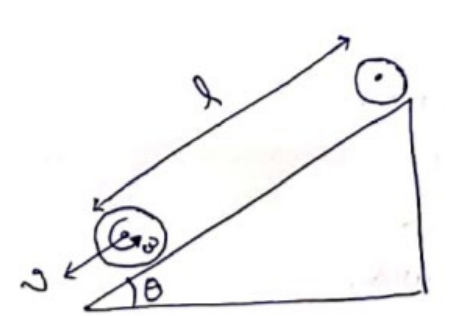# Solve the following :

Question:

A sphere starts rolling down an incline of inclination $\theta$. Find the speed of its centre when it has covered a distance $\mathrm{I}$.

Solution:By energy conservation,

$m g(l \sin \theta)=\frac{1}{2} I \omega^{2}+\frac{1}{2} m v^{2}$

$m g l \sin \theta=\frac{1}{2}\left(\frac{2}{5} m R^{2}\right)\left(\frac{v^{2}}{R^{2}}\right)+\frac{1}{2} m v^{2}$

$v=\sqrt{\frac{10}{7} g l \sin \theta}$